﻿ A New and Efficient Proposed Approach to Find Initial Basic Feasible Solution of a Transportation ProblemPublications are Open
Access in this journal
Article Versions
Export Article
• Normal Style
• MLA Style
• APA Style
• Chicago Style
Original Article
Open Access Peer-reviewed

### A New and Efficient Proposed Approach to Find Initial Basic Feasible Solution of a Transportation Problem

Opara Jude , Oruh Ben Ifeanyichukwu, Iheagwara Andrew Ihuoma, Esemokumo Perewarebo Akpos
American Journal of Applied Mathematics and Statistics. 2017, 5(2), 54-61. DOI: 10.12691/ajams-5-2-3
Published online: July 06, 2017

### Abstract

In this research, a new and efficient approach of finding an initial basic feasible solution to transportation problems is proposed. The proposed approach is named “Inverse Coefficient of Variation Method (ICVM)”, and the method is illustrated with seven numerical examples. Six existing methods; North West Corner Method (NWCM), Column Minimum Method (CMM), Least Cost Method (LCM), Row Minimum Method (RMM),Vogel’s Approximation Method (VAM), and Allocation Table Method (ATM) were compared with the proposed approach. It can be said conclusively that the proposed Inverse Coefficient of Variation Method (ICVM) provides an improved Initial Basic Feasible Solution to all the transportation problems used in the experiment. Further, the new method leads to the optimal solution to many of the problems considered.

### 1. Introduction

Transportation problem is popular in operations research for its real life wide application. This is a special kind of network optimization problems which deals with the determination of a minimum-cost schedule for transporting a single commodity from a number of sources (warehouses) to a number of destinations (markets). This class of problem which is basically a linear programming problem can be extended to some practical applications such as inventory control, staff assignment, job scheduling, cash flow etc. The transportation algorithm is based on the assumption that the model is balanced, meaning that the total demand equals the total supply. If the model is unbalanced 5, 13, we can always augment it with a dummy source or a dummy destination to restore balance.

The basic transportation problem was originally developed by Hitchcock in 1941. Efficient methods for obtaining solution were developed, primarily by Dantzig in 1951 3, followed by Charnes et al in 1953 2.

The optimal solution to the classical transportation problem requires the determination of a number of units of commodities to be transported from each origin to various destinations, satisfying source availability and destination demands so that the total cost of transportation is minimum. The available amounts at the supply points and the amounts required at the demand points are the parameters of the transportation problem 4.

The transportation problem has a lot of special structure. For instance, each variable appears in exactly two constraints (with a non-zero coefficient). When a variable has a non-zero coefficient, the coefficient is either plus or minus. Due to this special structure, two possible things turn out to be true. The first is that, there are alternative methods of solving transportation problems that are more efficient than the standard simplex algorithm. This turns out to be important in practice, because real-world transportation problems have enormous numbers of variables. The Second is that, because of the special structure, it is possible to solve the transportation problem in whole numbers. That is, if the data of the problem (supplies, demands, and costs) are all whole numbers, then there is a whole number solution. The significance of this property is that you do not need to impose the difficult to handle integer constraints in order to get a solution that satisfies the constraints.

There are basically three stages for the solution procedure for the transportation problem:

Stage 1: Mathematical formulation of the transportation problem,

Stage 2: Finding an initial basic feasible solution,

Stage 3: Optimize the initial basic feasible solution which is obtained in Stage 2.

Our focus in this study is on stage 2: obtaining an improved initial basic feasible solution for the transportation problems.

### 2. Related Literature Review

Megha et al 10 worked on a Comparative Study of Transportation Problem Using C++ and Mat-lab. The study was about solving transportation problem using Operation Research (OR) approach in analysis and design phases and they tried to use C++ programming language and Mat lab to model the problem. The results obtained from the method were compared in order to make analysis and prove the object-oriented model correctness. They tried to make the results obtained to be identical and have the same results when solving the problem using the five methods: Northwest Corner Method, Minimum Cost Method, Row Minimum Cost Method, Column Minimum Cost Method, and Vogel’s Approximation Method. The researchers recommended that in future, these problems should be compared for speed and accuracy using C + + and Mat-lab.

Opara et al 12 carried out an experimental study of the existing methods of solving linear transportation problems, comparing them with a new approach proposed by Mollah et al 11. The existing methods, North West Corner Method, Least Cost Method, and Vogel’s Approximation Method were compared to the newly proposed algorithm known as Allocation Table Method (ATM). Real life data were collected from Dangote Flour Mills Plc, Calabar, Cross River State Nigerian, and two numerical examples were collected from the exercise of Inyama 9. The Allocation Table Method and the three existing methods were used to solve the problems. From the analysis carried out in the study, it was observed that the allocation table method did not yield comparatively better result. Several number of cost minimizing transportation problems were solved using ATM and it was found that the Allocation Table Method did not yield better results compared to the existing methods. It was therefore recommended that Mollah et al 11 should re-visit their proposed algorithm to probably know the lacuna in it, and future researchers should also look into a similar study to make their comments as regards to this contradiction.

Abdul et al 1 worked on a comparative study of initial basic feasible solution methods for transportation problems. In their work, three methods were used to find an initial basic feasible solution for the balanced transportation model. They used a new method of Minimum Transportation Cost Method (MTCM) to find the initial basic feasible solution for the solved problem by Hakim 7. Hakim used Proposed Approximation Method (PAM) to find initial basic feasible solution for balanced transportation model and then compared the results with Vogel’s Approximation Method (VAM). The results of both methods were noted to be the same but in their study, they took the same transportation model and used MTCM to find its initial basic feasible solution and compared the result with PAM and VAM. It was noted that the MTCM process provided not only the minimum transportation cost but also an optimal solution.

Mollah et al 11 carried out a research on a New Approach to Solve Transportation Problems. Solution of transportation problems was proposed. Efficiency of allocation table method was tested by solving several number of cost minimizing transportation problems and it was found that the allocation table method yields comparatively a better result. Finally it can be claimed that the allocation table method may provided a remarkable Initial Basic Feasible Solution by ensuring minimum transportation cost. According to the researcher, it will help to achieve the goal to those who want to maximize their profit by minimizing the transportation cost.

Sankar and Gurupada 16 researched on minimizing cost and time through single objective function in multi-choice interval valued transportation problem. The study explored the study of Transportation Problem (TP) under the light of multi-choice environment with interval analysis. The parameters of TP followed multi-choice interval-valued type so the form of TP is called Multi-Choice Interval Transportation Problem (MCITP). Introduction of time was an important notion in TP of the paper. Transportation time and cost, both were minimized through single objective function of TP, which was the main aim of the study. A procedure was shown for converting from MCITP to deterministic TP and then solved it. A case study was included to illustrate the usefulness of the study. Finally, concluding remarks and an outlook for future study were presented to the study.

In the present work we experiment with a new transportation method that leads to an improved initial feasible solution to transportation problems.

### 3. Linear Transportation Model Problem

Transportation is an example of network optimization problem. It deals with the efficient distribution (transportation) of product (goods) and services from several supply locations (sources) with limited supply, to several demand locations (destinations) with a specified demand with the objective of minimizing total distribution cost.

This objective is achieved under the following constraints;

1. Each demand point receives its requirement.

2. Distributions from supply points do not exceed its available capacity.

This goal is achieved contingent on availability and requirements constraints. Transportation problem therefore assumes that the transportation cost on a given route is directly proportional to the number of units of the commodity transported 9.

### 4. Model Formulation

The formulation of the transportation model employs double – subscripted variables of the form xij. Thus, the general formulation of the transportation problem with supply (sp), demand (d), n sources and m destinations, is given by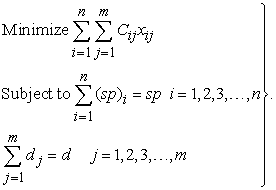The general formulation of the transportation problem reveals that m supply constraints and n demand constraints translate into m + n total constraints. The flow chart in Figure 1 illustrates the various phases leading to the optional solution of a transportation problem

### 5. Transportation Tableau

The transportation tableau is a unique tabular representation of the transportation problem. The xij variable gives the number of units transported from source i to destination j (which is to be solved for) while the unit cost for the transportation from i to j, denoted by Cij, is recorded in a small box in the upper – right – hand corner of each cell. Table A is the form of the general transportation tableau.

### 6. Methods for Finding Initial Basic Feasible Solution

The first phase of the algorithm for solving a transportation problem for optimal solution involves finding the initial basic feasible solution. An initial feasible solution is a set of arc flows that satisfies each demand requirement without supplying more from any origin node than the supply available. This paper compares only six heuristics method for developing an initial solution to transportation problem, with the new proposed approach; which are;

i. The Northwest Corner Method (NWCM)

ii. The Least Cost Method (LCM)

iii. The Vogel’s Approximation Method (VAM)

iv. Row Minimum Method (RMM)

v. Column Minimum Method (CMM)

vi. Allocation Table Method (ATM)

6.1. Algorithm for Inverse Coefficient of Variation Method (ICVM)

Our algorithm for the method for determining the initial basic feasible solution to transportation problem of the proposed approach is stated as follows:

Step 1: Construct a balance Transportation Table (TT) from the given transportation problem.

Step 2: Determine the mean (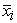),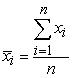and standard deviation (Si),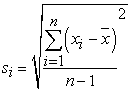for each row. Then obtain the respective inverse of coefficient of variation [(CV)-1],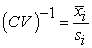Step 3: Determine the mean (),and standard deviation (Si),for each column. Then obtain the respectiveinverse coefficient of variation [(CV)-1],. Ignore any row or column whose standard deviation is zero.

Step 4: Identify the row or column with the smallest (CV)-1 among the rows and columns (breaking ties arbitrarily). Locate the cell with the lowest cost in the selected row or column and assign as many units as possible to it.

Step 5: Reduce the row supply and column demand by the number of units assigned to the cell and cross out the row supply or column demand that is satisfied then form a new tableau. If a row and a column are satisfied simultaneously, only one of them is crossed out and the remaining column (or row) is assigned a zero demand (or supply). Again, any column or row with zero demand or supply should not be used in computing subsequent(CV)-1.

Step 6: Recalculate the row and column (CV)-1 for the reduced transportation tableau, as indicated in steps 2 & 3, and then go to steps 4 & 5. Continue until all the requirements (demands and supplies) are satisfied.

Step 7: Finally calculate the total transportation cost of the TT. This calculation is the sum of the product of cost and corresponding assigned value of the TT.

6.2. Numerical Problems

We shall use seven numerical examples to illustrate the proposed algorithm. Examples one to three were extracted from Opara et al 12; examples four to six were gotten from Mollah et al 11; while the seventh example was gotten from Example 9.3 of Inyama 9.

Example 1

Consider a Transportation problem with four markets and four warehouses. The market demands are 10, 4, 6, and 14 while the warehouse capacities are 6, 9, 7, and 12. The cell entries represent unit cost of transportation, and the table is shown in Table 1.

We shall obtain the means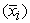, standard deviations (Si) and the inverse of the coefficient of variation (CV)-1 as shown in Table 2. For instance, to calculate the average, standard deviation, and (CV)-1 for warehouse one, we have,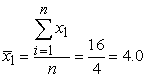,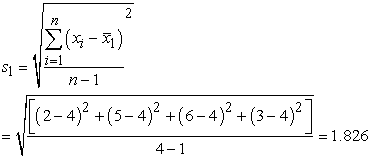and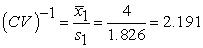The remaining warehouses 2, 3, and 4 were done in the same manner as well as markets 1, 2, 3, and 4 to obtain the averages, standard deviations and inverse coefficient of variations and the results are presented in Table 2. However, for easier computation, an electronic calculator or Microsoft excel can be used to compute, Si, and (CV)-1.

The minimum (CV)-1 is 1.217 which occurs in row 2. The least cost in this row is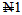which corresponds to column 4. Hence;

X24 = minimum (a2, b4) = minimum (9, 14) = 9. Row 2 is satisfied and crossed out, while column 4 remains 14 – 9 = 5. Thus, the summary of the adjustment is shown in Table 3.

The minimum (CV)-1 is 1.763 which occurs in column 3. The least cost in this column is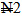which corresponds to row 4. Hence;

X43 = minimum (a4, b3) = minimum (12, 6) = 6. Column 3 is satisfied and crossed out, while row 4 remains 12 – 6 = 6. Thus, the summary of the adjustment is displayed in Table 4.

The minimum (CV)-1 is 1.855 (breaking ties arbitrarily) which occurs in column 1. The least cost in this column iswhich corresponds to row 1. Hence X12 = minimum (a1, b1) = minimum (6, 10) = 6. Row 1 is satisfied and crossed out, while column 1 remains 10 – 6 = 4. We now adjust the table to obtain the result in Table 5.

The minimum (CV)-1 is 1.273 which appears in column 2. The least cost in this column iswhich corresponds to row 3. Hence X32 = minimum (a3, b2) = minimum (7, 4) = 4. Column 2 is satisfied and crossed out, while row 3 is left with 7 – 3 = 3. We now adjust the table to get the result in Table 6.

The minimum (CV)-1 is 2.593 which appears in row 4. The least cost in this row is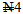which corresponds to column 4. Hence X44 = minimum (a4, b4) = minimum (6, 5) = 5. Hence column 4 is satisfied and crossed out, while row 4 is left with 6 – 5 = 1. We now adjust the table to get the result in Table 7.

The only (CV)-1remaining is 4.243 which appears in column 1 with a least cost of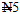, corresponding to row 3. Hence X31 = minimum (3, 4) = 3. Hence row 3 is satisfied and crossed out, while column 1 remains 4 – 3 = 1. After adjustment, only cell X41 will be left with a supply and demand of 1. This finally gives the summary result in Table 8.

Hence, the total transportation cost is 6(2) + 9(1) + 3(5) + 4(2) + 1(7) + 6(2) + 5(4) =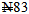.

We now improve the initial feasible solution obtained by first calculating the shadow costs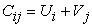where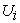and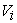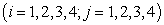are the multipliers. That is;

For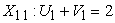For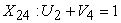For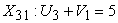For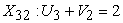For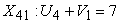For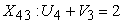For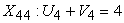Setting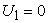and solving produces;,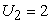,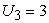,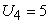;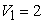,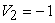,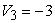,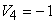.

We now proceed by calculating the potential benefits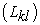of each of the 9 non-basic variables by using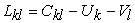. That is;

For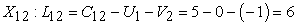For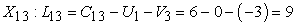For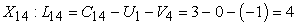For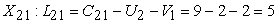For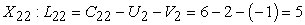ForFor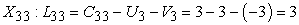ForFor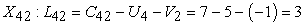Since none of the potential benefit isis negative, we stop; the present initial feasible solution is optimal. Hence the optimal solution is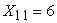,,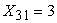,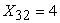,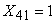,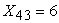,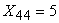and the minimum cost for this transportation problem is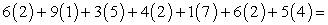.

If there is negative value in the potential benefits, then we select the cell with the most negative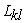value. The next step is assign the quantity “0” to the selected cell and find a group of cells which are presently in use (that is, variables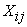) which when modified by the “0” value will keep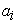and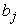values unchanged. In other words, a closed loop for the current entering variable will be constructed (the loop starts and ends with the entering variable). The loop consists of successive horizontal and vertical connected lines whose end points must be basic variables except the end points associated with the entering variable. The loop can be traced in clockwise or anti-clockwise direction for a given basic feasible solution; only one unique loop is possible for each non-basic variable. Then adjust the corner basic variable of the loop by 0. The last step is to set “0” to be as large as possible. Redraw tableau and go to the step of calculating the multipliersand. Hence, the method employed here to obtain the optimal solution is the Modified Distribution (MODI) Method.

The remaining six examples shall be presented in their last tableau without illustration.

Example 2

Dangote Flour Mills Plc is a manufacturing company located in Calabar. The company produces Bread Flour(BF), Confectionery Flour (CF), Penny Semolina (PS) and Wheat Offals (WO). These products are supplied to thefollowing states (locations) Bayelsa, Anambra, Rivers, Kano, Abia, Enugu, AkwaIbom etc. For the purpose ofthis study, only four (4) of these demand points shall be considered; Enugu, Akwa-Ibom, Anambra and Rivers. The estimated supply capacities of the four products, the demand requirements at the four sites (states) and the transportation cost per bag of each product are given in Table 9.

To obtain the initial basic feasible solution of Example 2, using the proposed algorithm, the final result is summarized in Table 10.

Hence, the total transportation cost is 12600(45) + 2900(52) + 9600(48) + 1400(56) + 1000(54) + 14400(58) + 11600(44) = 2,656,600.

Example 3

Consider a Transportation problem with four markets and three warehouses. The market demands are 10, 6, 8, and 12 while the warehouse capacities are 12, 14, and 10.The cell entries represent unit cost of transportation, and the table is shown in Table 11.

The final result using the proposed algorithm is displayed in Table 12.

Total transportation cost = 10(5) + 2(9) + 6(7) + 6(10) + 2(5) + 10(1) = 190.

Example 4

A company manufactures motor cars and it has three factories F1, F2 and F3whose weekly production capacities are 300, 400 and 500 pieces of cars respectively. The company supplies motor cars to its four showrooms located at D1, D2, D3 and D4 whose weekly demands are 250, 350, 400 and 200 pieces of cars respectively. The transportation costs per piece of motor cars are given in the transportation Table 13. Find out the schedule of shifting of motor cars from factories to showrooms with minimum cost:

The final result using the proposed algorithm is displayed in Table 14.

Total transportation cost = 300(1) + 250(2) + 150(5) + 50(3) + 250(3) + 200(2) = 2850.

Example 5

Consider the following transportation problem (Transportation Table 15) involving four sources and four destinations. The cell entries represent the cost of transportation per unit. Obtain an initial basic feasible solution.

The final result using the proposed algorithm is displayed in Table 16.

Total transportation cost = 5(7) + 5(5) + 20(9) + 25(3) + 20(3) + 5(2) + 10(3) = 415.

Example 6

Consider the following transportation problem (Transportation Table 17) involving three origins and three destinations. The cell entries represent the cost of transportation per unit. Obtain an initial basic feasible solution.

Using the proposed algorithm, the summary result is presented in Table 18.

The transportation case = 90(3) + 30(5) + 50(4) + 70(8) + 30(7) = 1390.

Example 7

Consider the transportation problem with three markets and four warehouses. The market demands are 16, 10, 14, while the warehouse capacities are 11, 12, 10, 7. The unit cost of transportation is as given in Table 19.

The initial basic feasible solution using the proposed algorithm is presented in Table 20.

Total transportation cost = 11(4) + 8(7) + 4(5) + 10(3) + 5(5) + 2(6) = 187.

6.3. Analysis of Result

Having obtained the initial basic feasible solution using the proposed method with seven numerical data examples, the gotten results are compared with the existing methods.

Looking at Table 21, the transportation cost for the seven numerical examples used in this study, North West Corner Method (NWCM), and Column Minimum Method did not provide any optimal result; Least Cost Method (LCM) and Row Minimum Method (RMM) provided only one optimal result; Vogel’s Approximation Method (VAM) and Allocation Table Method (ATM) provided two optimal results; while the Proposed Inverse Coefficient of Variation Method (ICVM) provided four optimal results. The remaining three examples for the proposed method which are not optimal are close to optimal. Thus, the proposed Inverse Coefficient of Variation Method (ICVM) provides comparatively a better initial basicfeasible solution than the results obtained by the traditional algorithms which are either optimal or near to optimal. It is obvious that the performance of the solution varies for other methods which may be applicable in the proposed method, because it is quite difficult to assume a particular method that will result in the best solution in all examples that may arise. However, on the average, some methods are far better than others, which the proposed method is one of them.

### 7. Conclusion

The primary aim of a transportation model is to provide a strong structure to obtain the best ways to deliver goods from sources to destinations (customers).In this study, a new approach named Inverse Coefficient of Variation Method (ICVM) for finding an initial basic feasible solution of a balanced transportation problem is proposed. Coefficient of Variation Inverse Method has been tested and proven efficient by solving several number of cost minimizing transportation problems and it is discovered that the Inverse Coefficient of Variation Method gives comparatively a better result. Conclusively, it can be said that Inverse Coefficient of Variation Method an improved Initial Basic Feasible Solution by ensuring minimum transportation cost, though it may be a bit greater in some examples, but the good thing for this proposed method is that in most cases, it yields an optimum or close to optimum solution. This study will assist to achieve the aim of maximizing profits by minimizing the cost of transportation. The method employed here to obtain the optimal solution is the Modified Distribution (MODI) Method.

Future study should look at similar research by comparing other existing methods which were not incorporated in this study to the proposed technique. Again, a program code should be written for the proposed technique for ease computation.

### References

  Abdul, S. S., Gurudeo, A. T., and Ghulam, M. B. (2014). A Comparative Study of initial basic feasible solution methods for transportation problems. Mathematical Theory and Modeling. Vol.4, No.1, 2014 In article View Article  Charnes, A., Cooper, W.W. and Henderson, A. (1953). An Introduction to Linear Programming. John Wiley & Sons,New York. In article PubMed  PubMed  Dantzig, G.B. (1951). Application of the Simplex Method to a Transportation Problem, Activity Analysis of Productionand Allocation. In: Koopmans, T.C., Ed., John Wiley and Sons, New York, 359-373. In article  Deshabrata, R. M., Sankar, K. R., Mahendra, P.B. (2013). Multi-choice stochastic transportation problem involving extreme value distribution. 37(4). In article View Article  Goyal, S.K. (1984). Improving VAM for unbalanced transportation problems, Journal of Operational Research Society, 35(12) (1984) 1113-1114. In article View Article  Gurupada, M., Sankar, K. R., and José, L. V. (2016). Multi-Objective Transportation Problem With Cost Reliability Under Uncertain Environment. International Journal of Computational Intelligence Systems, 9(5). In article View Article  Hakim, M.A. (2012). An Alternative Method to Find Initial Basic Feasible Solution of a Transportation Problem, Annals of Pure and Applied Mathematics, Vol. 1, No. 2, 2012, 203-209. In article View Article  Hitchcock, F.L. (1941). The Distribution of a Product from Several Sources to Numerous Localities. Journal of Mathematics and Physics, 20, 224-230. In article View Article  Inyama S.C. (2007): Operation Research: Introduction. Supreme Publisher, Owerri. In article PubMed  Megha, A. J., Madhu, S.P., and Ganthade, B.H. (2017). A comparative study of transportation problem using C++ and Mat lab. International Conference on Emanations in Modern Engineering Science and Management. Vol. 5, Issue 3. In article  Mollah, M. A., Aminur, R. K., Sharif, U., and Faruque, A. (2016). A New Approach to Solve Transportation Problems. Open Journal of Optimization, 2016, 5, 22-30. In article View Article  Opara, J., Okenwe, I., and Iheagwara, A.I. (2016). Comparison of Existing Methods of Solving Linear Transportation Problems with a New Approach. International Journal of Innovation in Science and Mathematics. Volume 4, Issue 5. In article  Ramakrishnan, G.S. (1988). An improvement to Goyal's modified VAM for the unbalanced transportation problem, Journal of Operational Research Society, 39(6) (1988) 609-610. In article View Article  Roy, S.K. (2016). Transportation Problem with Multi-choice Cost and Demand and Stochastic Supply J. Operations Research of China (2016) 4(2), 193-204. In article View Article  Roy, S.K., Maity, G. & Weber, G.W. (2017). Multi-objective two-stage grey transportation problem using utility function with goals Cent Eur. J. Oper. Res. (2017) 25: 417. In article View Article  Sankar, K. R. and Gurupada, M. (2017). Minimizing cost and time through single objective function in multi-choice interval valued transportation problem, Journal of Intelligent & Fuzzy Systems 32(3), 1697-1709. In article View Article  Sankar, K.R., Gurupada, M., Gerhard, W.W., Sirma, Z.A.G. (2016). Conic Scalarization Approach to solve Multi-choice Multi-objective Transportation Problem with interval goal. Annals of Operations Research, August 2016. In article View Article  Sudipta, M. & Sankar, K. R. (2014). Solving Single-Sink, Fixed-Charge, Multi-Objective, Multi-Index Stochastic Transportation Problem. American Journal of Mathematical and Management Sciences. 33(4). In article View ArticleThis work is licensed under a Creative Commons Attribution 4.0 International License. To view a copy of this license, visit http://creativecommons.org/licenses/by/4.0/